# Supply and Demand

• Supply and demand model

• a model of how a competitive market functions
• The demand curve

• The supply curve

• The determinants of demand and supply

• The equilibrium price and quantity

# Demand Schedule and Demand Curve

• The law of demand

• A higher price leads to a lower quantity demaned

• A lower price leads to a higher quantity demanded

• Demand schedule vs. demand curve

• A demand schedule is a table that shows the quantity demanded at each price.

• A demand curve is a graph that shows the quantity demanded at each price

• Demand vs. quantity demanded

demand the relationship between a range of prices and the quantities demanded at those prices, as illustrated by a demand curve or a demand schedule.
quantity demanded only a certain point on the demand curve or one quantity on the demand schedule
• Demand refers to the curve, and quantity demanded refers to a specific point on the curve.

• If price increases, what happens?

• No change in demand

• Decrease in quantity demanded

• Graphical Comparison• Demand curve

• Horizontal axis: Quantity Demanded

• Vertical axis: Price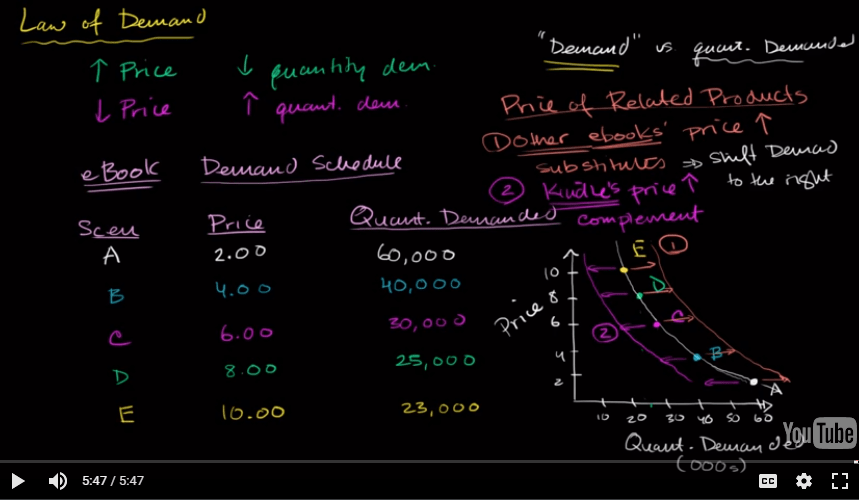# Shift of the Demand Curve

• Changes in the price of related goods

• Substitutes (positive correlation)

• fall in the price of one goods makes consumers less willing to buy the other good
• Complements (negative correlation)

• fall in the price of one of the goods makes consumers more willing to buy the other good
• How to remember

• Kids are positive when having a substitute teacher

• We lived in a cynical world. If someone gives you a complement, the true intention might be negative.

• Changes in income

• Normal goods

• rise in income increases demand

• ie. computers, Disneyland, steak

• Inferior goods

• rise in income decreases demand

• ie. macaroni & cheese, top ramen

• Normal goods vs. inferior goods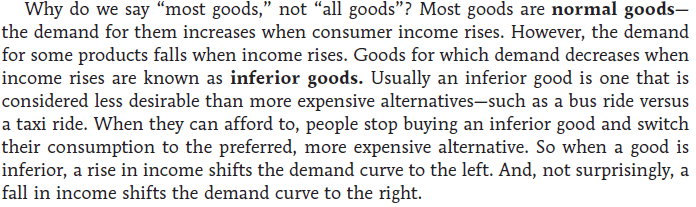• Changes in tastes

• Why do people want what they want?

• Changes due to fad, beliefs, cultural shifts are all clumped together under preferences.

• Changes in expectations

• If you expect more income in the future, demand for certain goods (ie. car or refrigerator) might increase

• If expectation of a future price drop of items exist, then the demand for these items drop today, almost like a self-fulfilling prophecy.

• Graph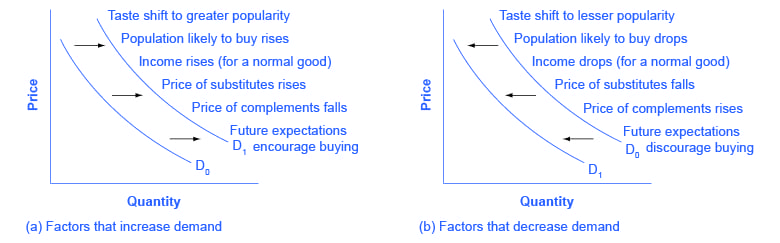• Summary# Supply Schedule and Supply Curve

• The law of supply

• A higher price leads to a higher quantity supplied.

• A lower price leads to a lower quantity supplied.

• Supply schedule vs. supply curve

• A supply schedule is a table that shows the quantity supplied at each price.

• A supply curve is a graph that shows the quantity supplied at each price.

• Supply vs. quantity supplied

supply the relationship between a range of prices and the quantities supplied at those prices, as illustrated by a supply curve or a supply schedule.
quantity supplied only a certain point on the supply curve or one quantity on the supply schedule
• Supply refers to the curve, and quantity supplied refers to a specific point on the curve.

• If price increase, what happens to supply?

• Nothing

• This is a change in quantity supplied not supply

# Shifts of the Supply Curve

• Changes in input prices

• Input is a good used to produce another good

• ie. cheese in a cheese pizza

• Change in technology

• All the ways in which people can turn more inputs into useful goods

• For example, an improved strain of corn resistant to disease increase supply of corn.

• Change in expectations

• If expectations of a future price increase of items exist, then supplier will tend to hoard the item in order to make more profit in the future.
• Related goods

• If the price of other things I can produce goes up, then my supply of grapes, once again, would go down.• Graph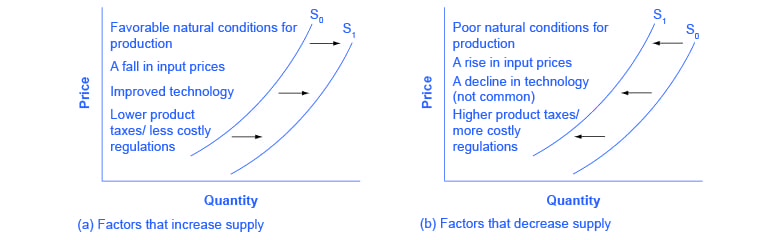• Summary# Supply, Demand, and Equilibrium

• Equilibrium price

• price that clears the market
• Equilibrium quantity

• quantity of good bought and sold at market-clearing price
• Equilibrium

• where the supply and demand curves intersect
• Graph# Surplus & Shortage

• Surplus

• when quantity supplied exceeds quantity demanded

•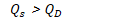•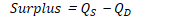• Shortage

• when quantity demanded exceeds quantity supplied

•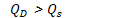•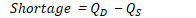• Graph